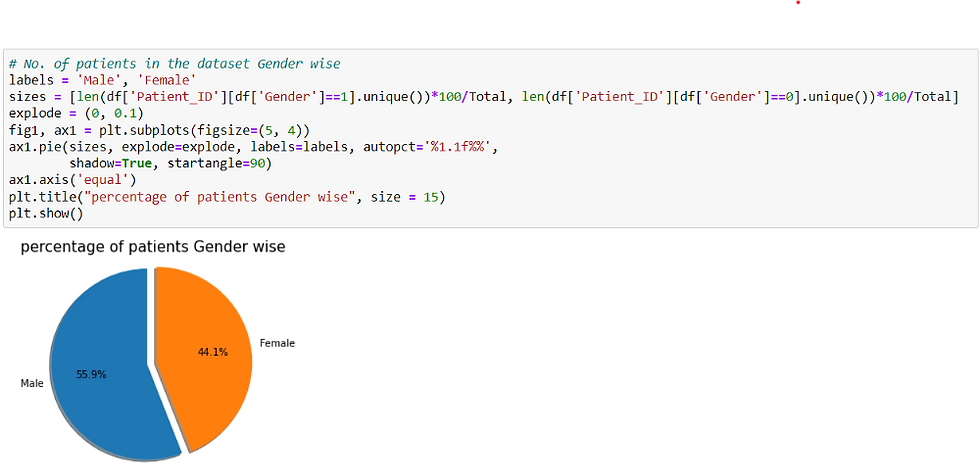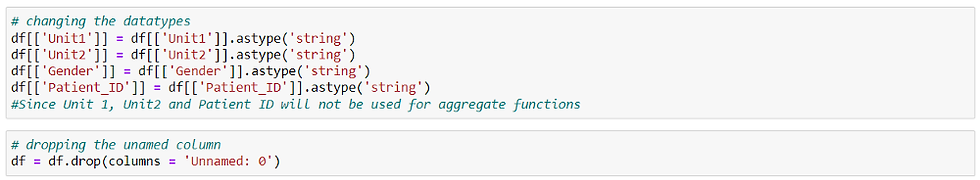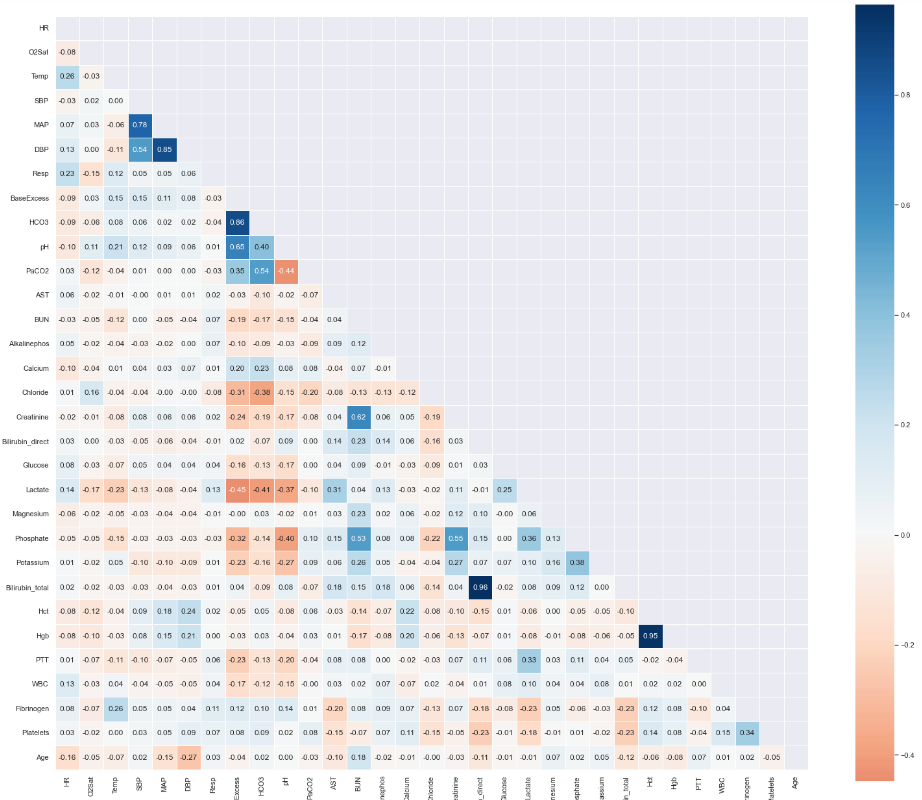top of pageSearch

# Explorative Data Analysis (EDA) of sepsis Dataset using Python

The purpose of this dataset is to train a machine learning model that can predict the presence of sepsis in patients, as well as to perform data analysis using tools such as Tableau or Power BI. The accuracy of the machine learning prediction algorithm is heavily influenced by the quality of the dataset used. Ensuring the availability of a high-quality dataset is the primary challenge in producing an accurate prediction. Sepsis is a life-threatening condition that occurs when the body overreacts to an infection, leading to damage to its own tissues. This condition can be caused by various types of viral or bacterial infections such as pneumonia or UTI.

What is EDA?

Exploratory Data Analysis (EDA) is an essential step in any data-related project, as it is used to gain a better understanding of the data. EDA involves generating summary statistics for numerical data in the dataset, and creating various graphical representations to identify patterns, outliers, and other important information about the data. By performing EDA, one can gain insights into the data such as its data types, missing values, skewness, and other statistical characteristics. This information is useful for data analysis and can also help to improve the accuracy of machine learning models, by identifying which features are most relevant for making predictions. Overall, EDA is a crucial step in the data analysis process, and it enables data scientists to make more informed decisions about how to proceed with their analyses.

understanding the data

This step involves importing the libraries like pandas, numpy, seaborn and matplot lib and loading the data into dataframe.The data consisted of a combination of hourly vital sign summaries, laboratory values, and patients’ demographics. Dataset contains 1552210 records with 43 features or fields.describe() method shows basic statistical characteristics of each numerical feature (int64 and float64 types): number of non-missing values, mean, standard deviation, range, median, 0.25, 0.50, 0.75 quartiles and for categorical features, count, unique, top (most frequent value), and corresponding frequency. This gives us a broad idea of our dataset.

### Features:

• Vital Signs : Heart Rate, Temperature , Blood Pressure, etc.

• Laboratory Values : Platelet Count, Glucose , Calcium etc.

• Demographics : Age, Gender, ICULOS, HospitalAdmtime

• SepsisLabel : 0 and 1

Data set has sepsis Label feature its value is either 0 or 1. The patients can be categorized under 3 conditions based on this feature.

Non sepsis Patients: NO sepsis on admission (Sepsis Label 0).

Sepsis after admission: No sepsis on admission, but later developed Sepsis in ICU (Sepsis Label 0 to 1).

Sepsis Patients are sum of the Patients Sepsis after admission and Sepsis before admission.There are 40,336 number of patients in the dataset and in that 92.7 % patients are Sepsis patients, 7.3% patients are Non sepsis.There are 55.9% Male patients and 44.1 % Female patients.

Cleaning the data:

Changing the datatype:Data types of Patient_ID, Unit1, Unit2,Gender are changed to string.

Unnamed: 0 column is dropped.

Finding the missing values :Lot of Numerical parameters in the dataset have missing values these can be imputed by front and back filling, filling with mean, filling with median, filling with mode, and mean-median filling. we can also drop the columns which have more percentage of null values by dropna() function.

Finding duplicate values:We can use duplicate.sum() function to find the sum of duplicate values. It will show the number of duplicate values if they are present in the data. This data does not have duplicate values.

Finding the correlation:

We can find the pairwise correlation between the different columns of the data using the corr() method.

The resulting coefficient is a value between -1 and 1 inclusive, where:

• 1: Total positive linear correlation

• 0: No linear correlation, the two variables most likely do not affect each other

• -1: Total negative linear correlation

Pearson Correlation is the default method of the function “corr”.

Now, we will create a heatmap using Seaborn to visualize the correlation between the different columns of our data:• Bilirubin_direct and Bilirubin_total are highly corelated and Bilirubin_direct is missing for about 96 % of patients. so,we can drop the Bilirubin_direct.

• DBP(diastolic BP),MAP (mean arterial pressure), and SBP (systolic BP) are highly corelated. MAP can be calculated from DBP and SBP, thus, we can disregard DBP and SBP.

• hemoglobin (Hgb) and hematocrit (Hct) values happened to be highly correlated. we can consider one feature, i.e hemoglobin (Hgb).

• pH, HCO3, and PaCO2 are also highly correlated. we can consider any one from these.

Finding the outliers:

Outlier of any feature can be found by following code:We can also use boxplot to visualize the outliers. A boxplot helps us in visualizing the data in terms of quartiles.As we can observe from the above boxplot that the normal range of data lies within the block and the outliers are denoted by the small circles in the extreme end of the graph.

So to handle it we can either drop the outlier values or replace the outlier values using IQR(Interquartile Range Method).

IQR is calculated as the difference between the 25th and the 75th percentile of the data. The percentiles can be calculated by sorting the selecting values at specific indices. The IQR is used to identify outliers by defining limits on the sample values that are a factor k of the IQR. The common value for the factor k is the value 1.5.

pH has outlier. These can be removed or replace them to the upper and lower limit based on its value. similar way we can check outliers for other numerical columns.

Conculsion:

EDA is crucial step it helps in better understanding of the problem statement and relationships between various features of the data. It involves a variety of graphical techniques to analyze the distribution of the data, the number of null values, and outliers.

References:

https://www.kaggle.com/code/namratakapoor1/sepsis-classification/data